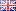# Forum: PC-Programmierung C++ program return an arrayAnnouncement: there is an English version of this forum on EmbDev.net. Posts you create there will be displayed on Mikrocontroller.net and EmbDev.net.

Lesenswert?
 • ▲ ▼
Hey guys
I am new with c++ and I have to write a code, but somehow it is not
working and I do not know what I can change. I would like to give back
the array Grad, but it says segmentation fault (core dumped).
Could somebody help me what I have to change?
Or is it possible to have a global variable and write from the function
directly to the global variable?

Code:
double* Gradient(const double x[], int n1) {
// We calculate the overlap. If the overlap is zero, the gradient
// is also zero. This should be probably improved in the future.
//int n = sizeof(x)/32;
for (int i = 0; i < n1*4; i++)
{
for (int j = 0; j < n1*4; i++)
{
if (i != j)
{
//cout << x[i]<< endl;
double xmin = max(x[i*4], x[j*4]);
double xmax = min(x[i*4+1], x[j*4+1]);
//if (xmin > xmax) return Grad;
double ymin = max(x[i*4+2], x[j*4+2]);
double ymax = min(x[i*4+3], x[j*4+3]);
//if (ymin > ymax) return Grad;

double x_overlap = xmax-xmin;
double y_overlap = ymax-ymin;

if (x[i*4] >= x[j*4] && x[i*4] != x[j*4+1] && x[i*4+1]
!= x[j*4] && x[i*4+2] != x[j*4+3] && x[i*4+3] != x[j*4+2]) Grad[i*4] =

y_overlap;

if (x[i*4+2] >= x[j*4+2] && x[i*4] != x[j*4+1] &&
x[i*4+1] != x[j*4] && x[i*4+2] != x[j*4+3] && x[i*4+3] != x[j*4+2])

x_overlap;

// Gradient for xmax if rectangles are touching by the
x-coordinate
if (x[i*4+1] == x[j*4] && x[i*4+3] > x[j*4+2] &&
x[i*4+2] < x[j*4+3])
{
}

// Gradient for ymax if rectangles are touching by the
y-coordinate
if (x[i*4+3] == x[j*4+2] && x[i*4+1] > x[j*4] && x[i*4]
< x[j*4+1])
{
}
}
}
}
}

int main() {
// Coordinates of the rectangles
double x[] = {0,6,0,9,3,9,4,11};
int n1 = sizeof(x)/32;

}

Lesenswert?
 • ▲ ▼
Hi,

I haven't read through all of your code yet but there are two
conceptional problems within the first two lines already. ;)

Code:
double* Gradient(const double x[], int n1) {

1) You cannot define an array using a variable for its size. It has to
be constant.

These two ways are allowed:
double grad; // or use a named constant, e.g. MY_ARRAY_SIZE

while this one is not:
int size=5;

function and let it return a pointer to that array. Unfortunately,
This is probably, what causes the segfault.

If you really HAVE TO use a variable array size (Which doesn't make much
sense on a µC btw.) then you should have a look at 'new' and 'delete'
which corresponds to malloc/free in C.

This procedure is called: CallByReference.

hth

Lesenswert?
 • ▲ ▼
Moritz O. schrieb:
> Hey guys
> I am new with c++ and I have to write a code, but somehow it is not
> working and I do not know what I can change. I would like to give back
> the array Grad, but it says segmentation fault (core dumped).

You should crank up the warning settings of your compiler. There are
multiple issues that the compiler should have warned about. There should
also be multiple errors, so I don't know how you got your compiler to
accept that code.

> Could somebody help me what I have to change?

The problem is that you return a pointer to a local array. The variable
Grad exists only as long as the function Gradient is running. But you
return a pointer to it, so in main(), you are accessing a pointer to a
non-existant object.

> Or is it possible to have a global variable and write from the function
> directly to the global variable?

It is possible, but not a good idea.

Lesenswert?
 • ▲ ▼
Moritz O. schrieb:
> Or is it possible to have a global variable and write from the function
> directly to the global variable?

There is a way in the middle.

Define the array in the caller function (in your example: in main) and

(strcpy, fgets and other standard functions do this also)

Lesenswert?
 • ▲ ▼
Thank you very much guys :-).
I will try to apply this methods :-).

### Antwort schreiben

Die Angabe einer E-Mail-Adresse ist freiwillig. Wenn Sie automatisch per E-Mail über Antworten auf Ihren Beitrag informiert werden möchten, melden Sie sich bitte an.

### Wichtige Regeln - erst lesen, dann posten!

• Groß- und Kleinschreibung verwenden
• Längeren Sourcecode nicht im Text einfügen, sondern als Dateianhang

### Formatierung (mehr Informationen...)

• [c]C-Code[/c]
• [code]Code in anderen Sprachen, ASCII-Zeichnungen[/code]
• $Formel in LaTeX-Syntax$
• [[Titel]] - Link zu Artikel
• Verweis auf anderen Beitrag einfügen: Rechtsklick auf Beitragstitel,
"Adresse kopieren", und in den Text einfügen
 Name: E-Mail (wird nicht angezeigt): Betreff: Ähnliche Beiträge werden gesucht... Dateianhang: Bitte das JPG-Format nur für Fotos und Scans verwenden! Zeichnungen und Screenshots im PNG- oderGIF-Format hochladen. Siehe Bildformate. Hinweis: der ursprüngliche Beitrag ist mehr als 6 Monate alt.Bitte hier nur auf die ursprüngliche Frage antworten, für neue Fragen einen neuen Beitrag erstellen. Text: Mit dem Abschicken bestätigst du, die Nutzungsbedingungen anzuerkennen.